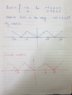# Sketching the graph of a function

Setareh7796
Homework Statement:
Sketch f(x) in the range -2l<x<2l
Relevant Equations:
The equation is in the picture attached
I have no idea why my sketch is wrong.

#### Attachments

•Function.jpg
35.9 KB · Views: 254

Mentor
But your function looks to be only defined over -l to +l -- why are you asked to extend it outside of that definition? Is there more to the question? Is that really how the problem is stated in the book?

Setareh7796
But your function looks to be only defined over -l to +l -- why are you asked to extend it outside of that definition? Is there more to the question? Is that really how the problem is stated in the book?

I have attached the full question as stated in the problem sheet. I am just stuck in the sketching part.

#### Attachments

Mentor
I have attached the full question as stated in the problem sheet.
Ah, yes. You left out the "and" part...So the period of the waveform is 2l, right? So f(x) should equal f(2l), not f(3l/2)...

Mentor
So f(x) should equal f(2l), not f(3l/2)...
According to the belated problem statement, f(x) = f(x + 2l).

Mentor
According to the belated problem statement, f(x) = f(x + 2l).
Yeah, thanks Mark. I meant f(x) = f(x + 2l), not = f(x + 3l/2). Trying to type too fast I guess...

Staff Emeritus
Homework Helper
Gold Member
That " l ", lower case L, sure makes these expressions difficult to read. There is a Unicode character that will help, Unicode character U + 2113, which is rendered as .

f(x) = f(x + 2l) becomes f(x) = f(x + 2ℓ)

##\LaTeX##, can also give a good result. Its standard use of a serif font in italics for variables gives ##f(x) = f(x + 2l) ##.

But the script lowercase L can be displayed in ##\LaTeX##. Use "\ell" to get ##f(x) = f(x + 2\ell) ##.

Now I must say that "\ell" looks better written with ##\LaTeX## as \##ell##.